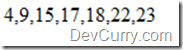# Sort Numeric Array in JavaScript

 Tweet

A lot of developers get confused with the results while calling the JavaScript sort() method on a numeric array. Let us see an example:

On running this code:

`<script type="text/javascript">    var arrNum = [23, 15, 17, 4, 9, 18, 22];    document.write("<p>" + arrNum.sort() + "</p>");</script>`

you get the following output:

This result is not what you expected right? What is happening here is that the elements are converted to their string equivalent before sorting. Hence the sort is not numerical.

To sort the numeric array, you will need to write a custom sort function as shown below:

`<script type="text/javascript">    var arrNum = [23, 15, 17, 4, 9, 18, 22];    function sortNumber(num1, num2) {        return num1 - num2;    }       document.write("<p>" + arrNum.sort(sortNumber) + "</p>");</script>`

The custom function sortNumber() sets the sort index of the parameter passed to it. So if num1 is less than num2, num2 has a higher sort index. If num1 is greater than num2, num1 will have a higher sort index and so on.

The result after sorting is as shown below: